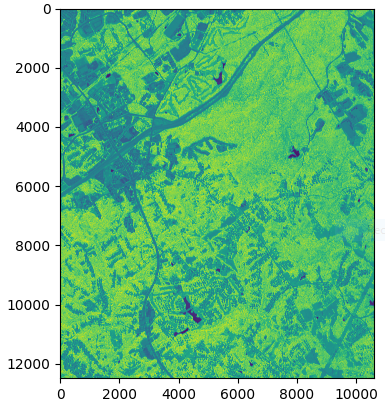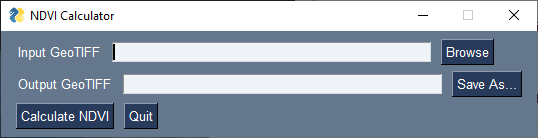# Quiz: Normalized difference vegetation index in Python

In this quiz, you want to develop an open-source Python application that is capable of calculating the normalized difference vegetation index (NDVI). This application should take one 4-band (R, G, B, and NIR) GeoTIFF file, calculate its NDVI, and save it as a GeoTIFF file. For testing, use m_3408350_nw_17_060_20191023.tif. Submit your Python script in FirstLastname_ndvi.py.

Allowed modules:

Penalties:

• Filename: -5
• Non-allowed modules: -5 each

Grading: -3 per debugged line up to the total points per question

## 1   Read the red and NIR bands of a GeoTIFF file as NumPy arrays

16 points

Using the GDAL module, open band 1 and read its content as an array into r. Repeat this process using band 4 for nir.

## 2   Normalize the red and NIR arrays

16 points

Normalize the r and nir arrays such that they range from 0 to 1.

## 3   Calculate the NDVI

16 points

Using the following equation, calculate ndvi. $\text{NDVI} = \frac{\text{NIR} - R}{\text{NIR} + R}$

## 4   Save the NDVI as a single-band GeoTIFF file

16 points

Using the GDAL module, save the ndvi array as a single-band GeoTIFF file into C:\tmp\ndvi.tif. The output file should properly be georeferenced using the geotransform matrix from the input GeoTIFF file.

## 5   Plot the NDVI

16 points

Using the matplotlib.pyplot submodule, plot ndvi.## 6   Create a GUI

20 points

Using the PySimpleGUI module, create a GUI for your NDVI calculator. Remove any hard-coded input and output file paths for any previous questions.## 7   Solutions

If you cannot solve any specific questions, you may use my solution files and move to the next one. In this case, you’ll lose the total points for the skipped question(s). To avoid downloads by mistake, you should’ve received the download password. There will be no excuse for downloading any solutions by accident.

Questions skippedLoss of pointsSolution